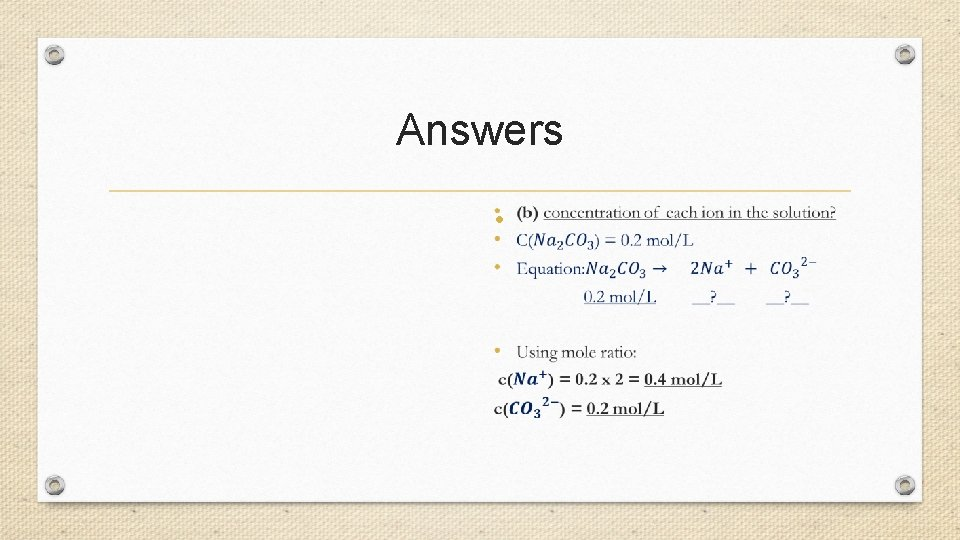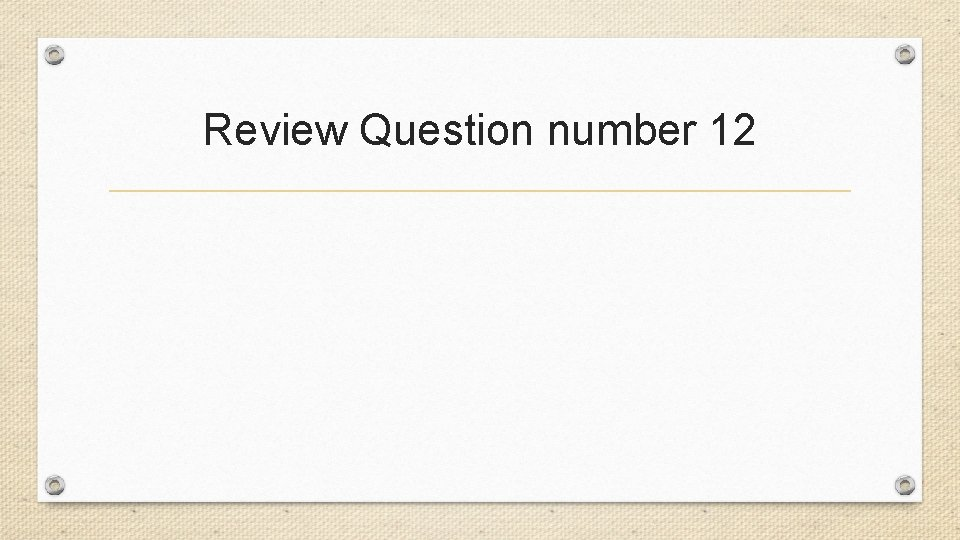# Stoichiometry Calculations involving balanced chemical equations Balancing Chemical

• Slides: 28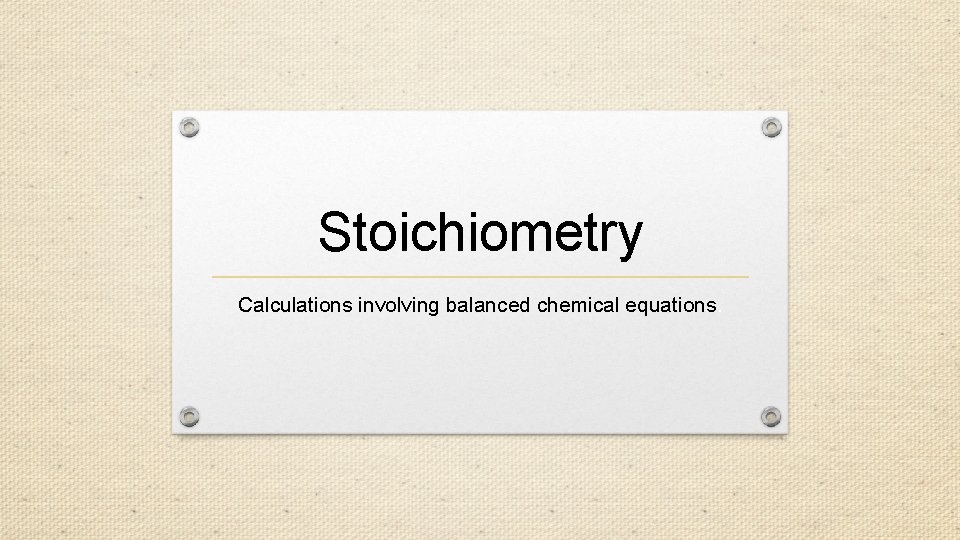Stoichiometry Calculations involving balanced chemical equations.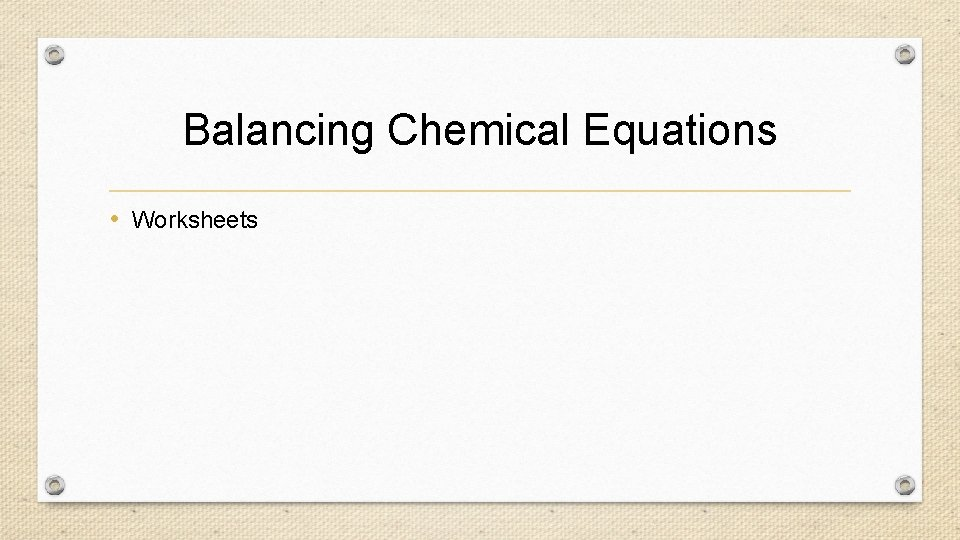Balancing Chemical Equations • WorksheetsA chemical equation tells us…. •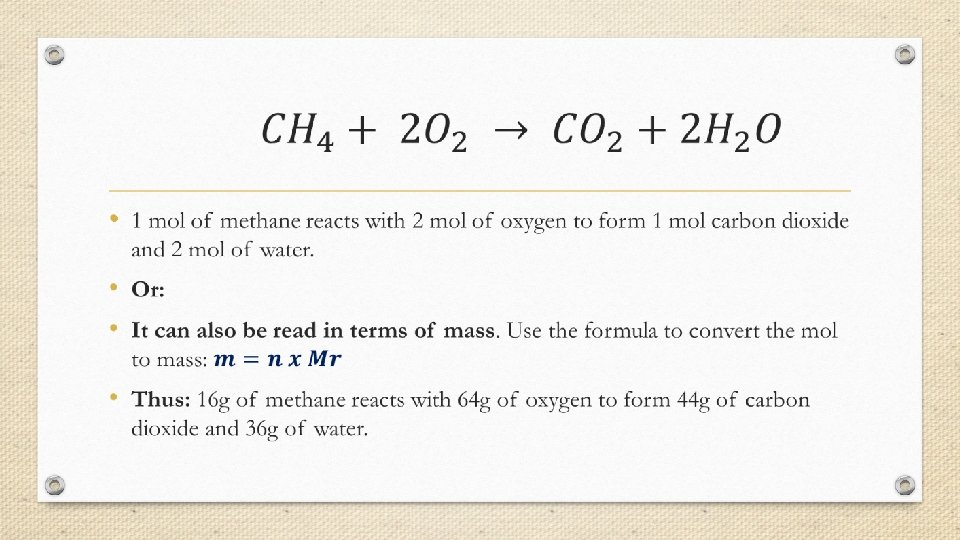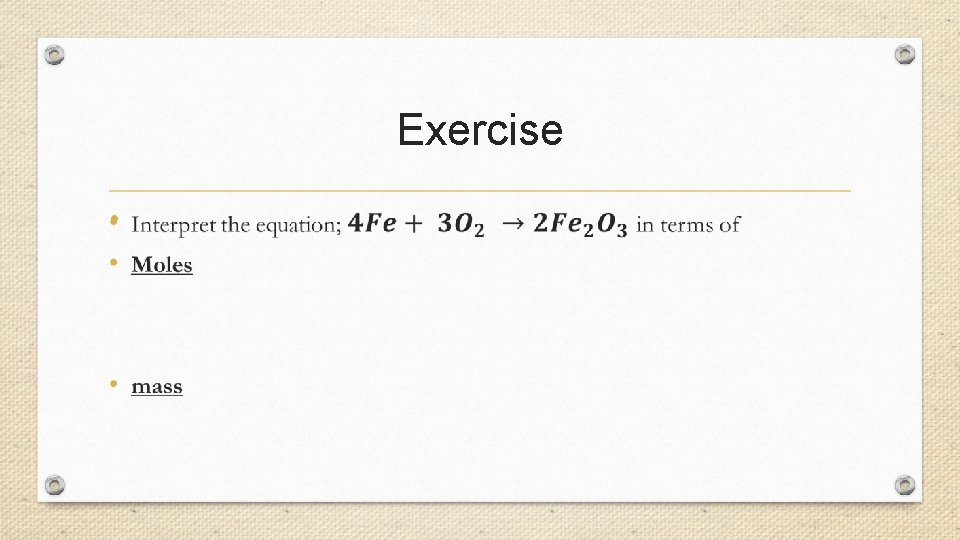Exercise •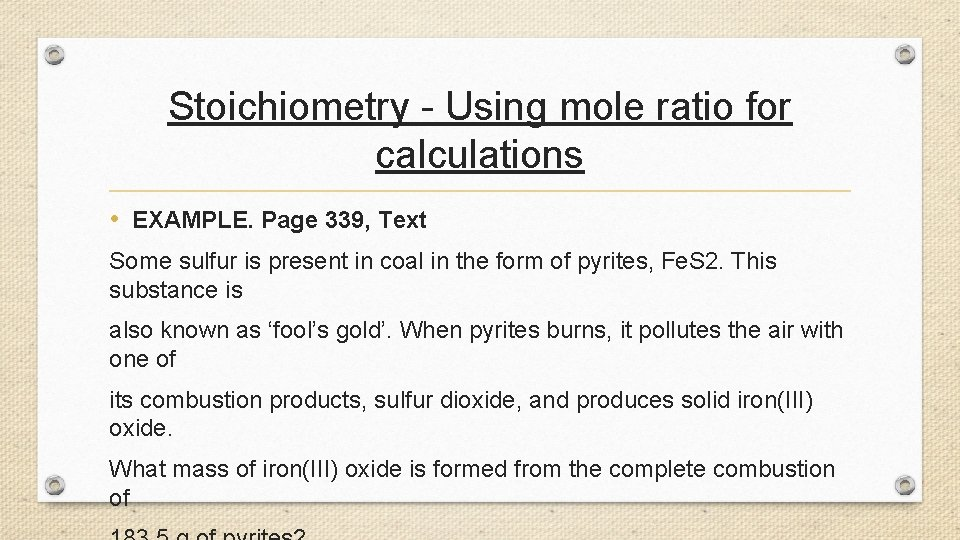Stoichiometry - Using mole ratio for calculations • EXAMPLE. Page 339, Text Some sulfur is present in coal in the form of pyrites, Fe. S 2. This substance is also known as ‘fool’s gold’. When pyrites burns, it pollutes the air with one of its combustion products, sulfur dioxide, and produces solid iron(III) oxide. What mass of iron(III) oxide is formed from the complete combustion ofTry the review questions on page 339 • Numbers 3, 5 and 63. Calculate the mass of water that is produced when 2. 8 g of methane is burnt in air. (Write a balanced equation first)Limiting Reagent/Reactant When certain amounts (moles) of reactants react together, they are combining and hence are they are getting used up. The reactant that gets completely used up first (finishes first) is known as the limiting reagent and hence stops the reaction from proceeding further. The moles of limiting reactant are used to do mole ratios for calculations as they determine the amount of products forming as they get used up first. The other reactant (that still has amounts left) is called the excess reactant.Exercise Magnesium and oxygen react to form magnesium oxide according to the following equation: If 24. 31 g of magnesium and 20. 00 g of oxygen are available for reaction, a) determine the limiting reactant; b) the reactant in excess and by how many mole c) and the mass of magnesium oxide formed.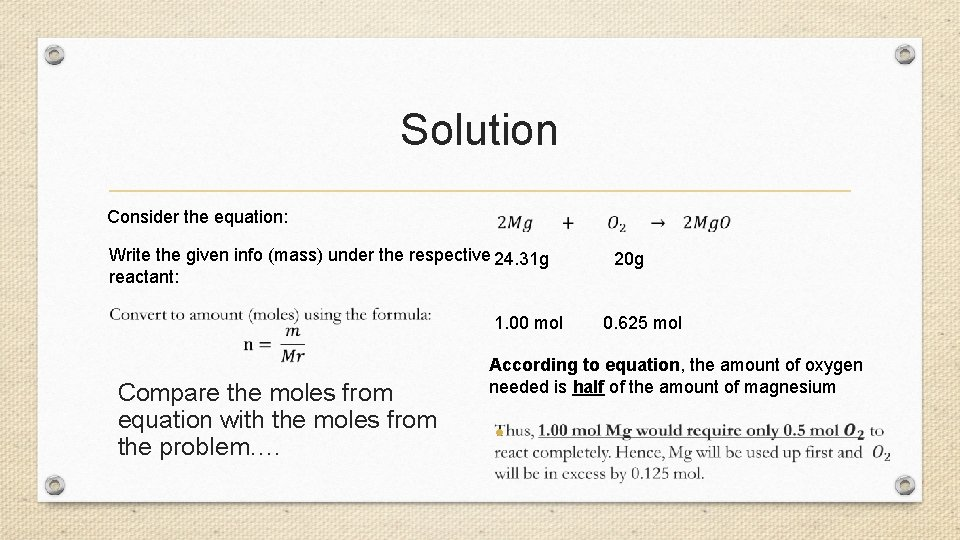Solution Consider the equation: Write the given info (mass) under the respective 24. 31 g 20 g reactant: 1. 00 mol 0. 625 mol Compare the moles from equation with the moles from the problem…. According to equation, the amount of oxygen needed is half of the amount of magnesium •Solution •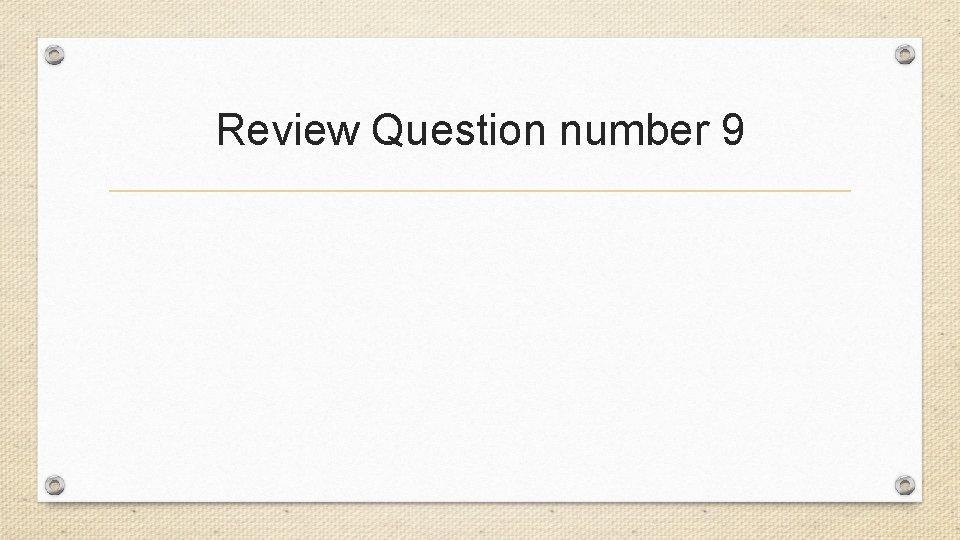Review Question number 9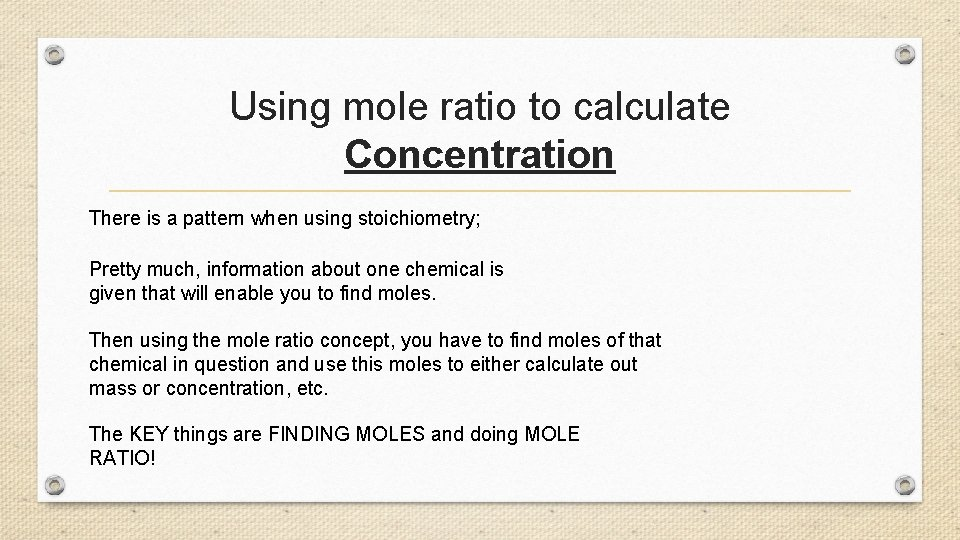Using mole ratio to calculate Concentration There is a pattern when using stoichiometry; Pretty much, information about one chemical is given that will enable you to find moles. Then using the mole ratio concept, you have to find moles of that chemical in question and use this moles to either calculate out mass or concentration, etc. The KEY things are FINDING MOLES and doing MOLE RATIO!Formulas…. •Examples to find Concentration • Calculate the number of moles of sodium chloride needed to prepare 500 m. L of a 0. 0800 mol L– 1 salt solution. What mass of sodium chloride would be weighed out? • Think about it……the concentration has to be 0. 08 mol/L…. . 0. 08 mol in 1 litre…this means in 500 ml, it would be 0. 04 mol Na. Cl…. . So how can we measure this amount and dissolve it to make a 500 ml solution which has a concentration of 0. 08 M? ?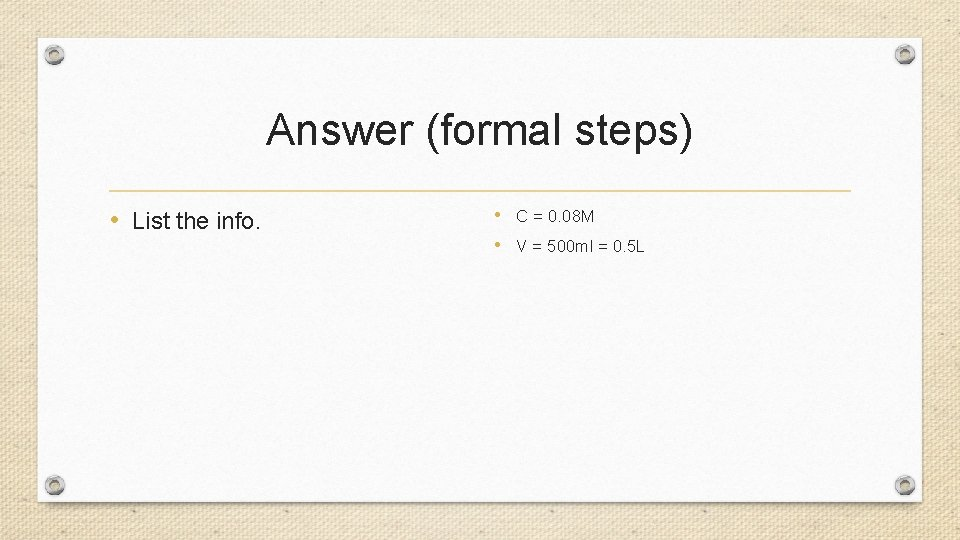Answer (formal steps) • List the info. • C = 0. 08 M • V = 500 ml = 0. 5 L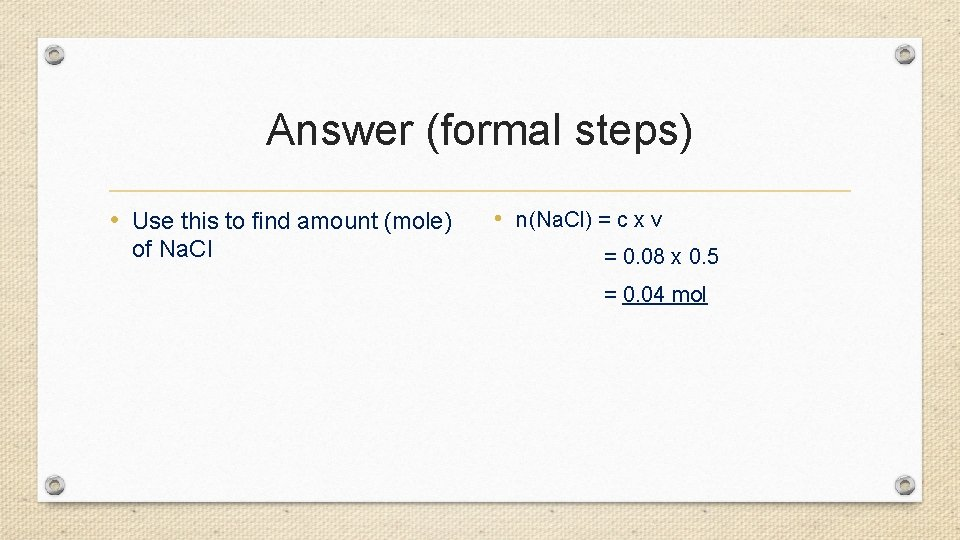Answer (formal steps) • Use this to find amount (mole) of Na. Cl • n(Na. Cl) = c x v = 0. 08 x 0. 5 = 0. 04 molAnswer (formal steps) • Work out the mass of Na. Cl • m(Na. Cl)= n x Mr = 0. 04 x 58. 5 = 2. 34 gReview Question number 10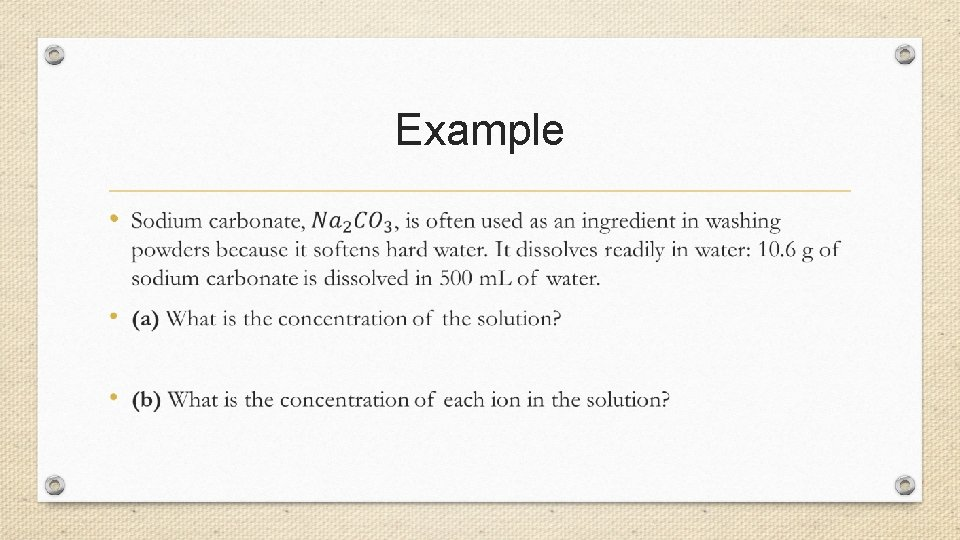Example •